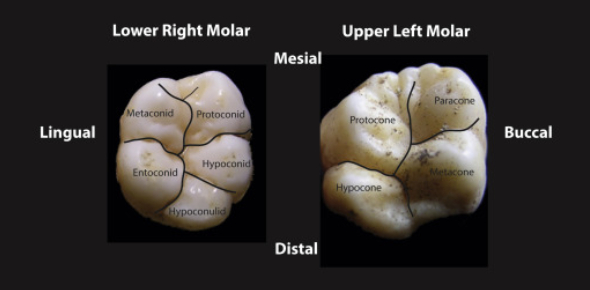Tooth Morphology Final Review

32 Questions | Total Attempts: 1718Settings.

• 1.
Which mandibular premolar is closest in form and function to a canine?
• A.

Tooth # 21

• B.

Tooth # 22

• C.

Tooth # 29

• D.

Tooth # 20

• 2.
On the mandibular first premolar, when looking at the occlusal outline of the buccal surface, which slope is shorter?
• A.

Mesio-occlusal

• B.

Disto-occlusal

• 3.
On which of the following teeth is there a mesiolingual developmental groove that originates in the mesial pit and continues onto the mesial surface?
• A.

Maxillary first premolar

• B.

Maxillary second premolar

• C.

Mandibular first premolar

• D.

Mandibular second premolar

• 4.
On the mandibular first premolar, there are how many cusps? How many are functional?
• A.

2 cusps, both are functional

• B.

2 cusps, only the buccal cusp is functional

• C.

2 cusps, only the lingual cusp is functional

• D.

3 cusps, only the buccal cusp is functional

• 5.
The mandibular second premolar has two lingual cusps, and the distolingual cusp is wider and longer.
• A.

True

• B.

False

• 6.
On the mandibular second premolar, the main groove pattern on the three cusp type takes the form of a...
• A.

U

• B.

Y

• C.

C

• D.

H

• 7.
Which two teeth are more alike in size, shape and function?
• A.

1st and 2nd maxillary premolars

• B.

1st and 2nd mandibular premolars

• 8.
In the maxillary arch, which tooth is larger?
• A.

Maxillary first premolar

• B.

Maxillary second premolar

• 9.
In the mandibular arch, which tooth is larger?
• A.

Mandibular first premolar

• B.

Mandibular second premolar

• 10.
Which ________ premolars crown dimensions are approximately equal, the __________ premolars are wider buccolingually than mesiodistally.
• A.

Maxillary, mandibular

• B.

Mandibular, maxillary

• C.

First, second

• D.

Second, first

• 11.
Which premolar is most likely to exhibit two root branches?
• A.

Mandibular first premolar

• B.

Mandibular second premolar

• C.

Maxillary first premolar

• D.

Maxillary second premolar

• 12.
The occlusalcervical dimension of the crown of this premolar is less than any anterior tooth but greater than any other premolar.
• A.

Mandibular first premolar

• B.

Mandibular second premolar

• C.

Maxillary first premolar

• D.

Maxillary second premolar

• 13.
Which of the following premolars exhibits 3 pits?
• A.

Mandibular first premolar

• B.

Mandibular second premolar (Y type)

• C.

Mandibular second premolar (U type)

• D.

Maxillary first premolar

• 14.
Which tooth has the most complex groove pattern?
• A.

Mandibular 3rd molar

• B.

Mandibular 2nd molar

• C.

Mandibular 1st molar

• 15.
The linguogingival groove is more commonly seen on which tooth?
• A.

Maxillary lateral

• B.

Maxillary central

• C.

Mandibular lateral

• D.

Mandibular central

• 16.
At what age should a child have all of their deciduous teeth?
• A.

3 years old

• B.

2 years old

• C.

6 months old

• D.

6 years old

• 17.
At what age do the deciduous teeth start being replaced by permanent teeth?
• A.

6 years old

• B.

7 years old

• C.

8 years old

• D.

9 years old

• 18.
On the deciduous maxillary canine, which cusp ridge is longer?
• A.

Mesial

• B.

Distal

• 19.
On the deciduous mandibular canine, which cusp ridge is longer?
• A.

Mesial

• B.

Distal

• 20.
The deciduous first maxillary molar has a transverse and oblique rige.
• A.

True

• B.

False

• 21.
On which tooth is the apex of the mesial root normally flat?
• A.

Permanent mandibular first molar

• B.

Permanent mandibular second molar

• C.

Primary mandibular first molar

• D.

Primary mandibular second molar

• 22.
Permanent First mandibular molars typically have how many cusps?
• A.

3

• B.

4

• C.

5

• 23.
Deciduous mandibular first molars have one transverse ridge.
• A.

True

• B.

False

• 24.
Which molar has the least complicated design of any molar?
• A.

Mandibular first molar

• B.

Maxillary first molar

• C.

Mandibular second molar

• D.

Maxillary second molar

• 25.
Which is the most common specimen of a mandibular third molar?
• A.

6 cusp pattern, 2 roots

• B.

5 cusp pattern, 2 roots

• C.

5 cusp pattern, 3 roots

• D.

4 cusp pattern, 2 roots

Related TopicsBack to top